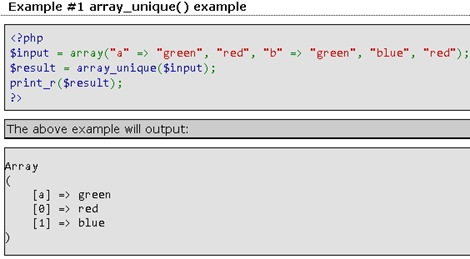# Php array unique

array array_unique ( array \$array [, int \$sort_flags = SORT_STRING ] )

Takes an input array and returns a new array without duplicate values.Note that keys are preserved. array_unique() sorts the values treated as string at first, then will keep the first key encountered for every value, and ignore all following keys. It does not mean that the key of the first related value from the unsorted array will be kept.

Note: Two elements are considered equal if and only if (string) \$elem1 === (string) \$elem2. In words: when the string representation is the same. The first element will be used.

Example #1 array_unique() example

`<?php \$input = array("a" => "green", "red", "b" => "green", "blue", "red"); \$result = array_unique(\$input); print_r(\$result); ?>`

The above example will output:

```Array
(
[a] => green
 => red
 => blue
)```

Example #2 array_unique() and types

```<?php \$input = array(4, "4", "3", 4, 3, "3"); ```

``` \$result = array_unique(\$input); var_dump(\$result); ```

`?>`

The above example will output:

```array(2) {
 => int(4)
 => string(1) "3"
}```

http://php.net/manual/en/function.array-unique.php